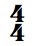top of page
Search
• by Mary Barton

# Excuse Me, Have You Got The Time …. Signature?

Updated: Feb 10, 2021One of the most fundamental skills we learn early in life is how to tell time. How to read a clock. In music, understanding the Time Signature is also a basic, fundamental skill and is as simple as telling time.

In the last post we learned that measures clarify the music visually and gather the notes together into equal bunches according to the basic rhythmic structure of the music. Time signatures tell us, at a glance, three things to expect in each measure:

1. The number of beats

2. The value of each beat

3. Where the main stress or stresses are

Let’s examine each of these.

1. The number of beats in each measure.

That’s the top number of the time signature. For example:= 2 beats per measure= 3 beats per measure= 4 beats per measure

And so on. It can’t get any simpler than that!

2. The bottom number tells us the value of each beat. While this is not complicated, we do need to learn a bit about different beat values in order to understand what the time signature is talking about!

Music is very mathematical. The most basic beat value is the quarter note:All other beat or note values are either fractions or multiplications of it. For example, we also have the eighth note:How many eighths fit into a quarter? Just as in math, two:We also have the sixteenth note:And again, as in math, we know that there are four sixteenths in a quarter:But we can also multiply the quarter note. For example, the half note:There are two quarters in a half:As I am sure you have guessed by now, there are other note values, but these are some of the most basic and common note values that you will encounter.

So, when we read a time signature, 3/4 time for example, we know that there are three quarter notes or the mathematical equivalent of three quarter notes in each measure. A measure in 3/4 time might look like one of these:(Note that when we have more than one 8th note on the same beat, they are joined together for better visual clarity. Same with the 16th notes below.)So, the top number indicates the number of beats; the bottom number, the value of each beat:= 2 quarter notes (or mathematical equivalent) per measure= 4 quarter notes (or mathematical equivalent) per measure= 3 eighth notes (or mathematical equivalent) per measure= 3 sixteenth notes (or mathematical equivalent) per measure

And so on! There are many possibilities.

3. And finally, the time signature tells us where the main stress goes.

Generally, in Western music, the strongest stress is on the first beat.= Strong-weak.= Strong-weak-weak. That’s your waltz rhythm.= Strong - weak-medium - weak.All the above time signatures are called simple time. But there are also compound time signatures which group the notes already gathered into the measures into even smaller bunches within the measure itself, creating patterns of stresses. For example, 6/8 time has six eighth notes (or the mathematical equivalent thereof) in each measure, but they are grouped in three’s within the measure itself:In 6/8 time, the first note of the first group gets the strongest stress, and the first note of the second group gets a medium stress:Sometimes the rhythmic structure of a music piece or the spoken language of the composer can alter which beat normally gets the strongest emphasis in a particular time signature (for example, some languages emphasize the second syllable of words). You will learn how to discern and navigate exceptions as you encounter a variety of repertoire with a reliable teacher.

There are many more simple and compound time signatures, but this gives you an idea of how time signatures work.

Of course, this raises the question, how long or fast is a quarter note? How short is an eighth note? How do we play these?

Stay tuned! We’ll discover that next time! As usual, it’s easier than you think!